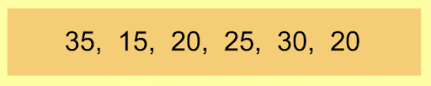Finding the Range 1
Set 1 (10 Questions)
Students collect numerical data and record, organize, display, and interpret the data on bar graphs and other representations:
Record numerical data in systematic ways, keeping track of what has been counted.
Identify features of data sets (range and mode).
From Mr. Anker Tests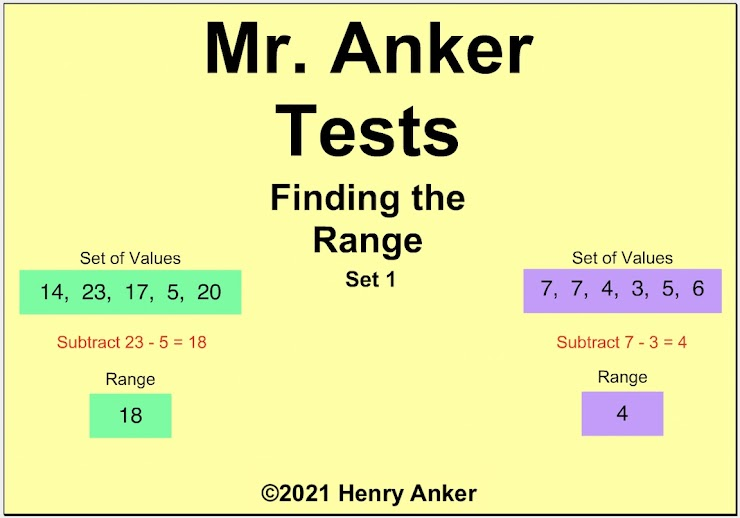Finding the Range Help Slide 1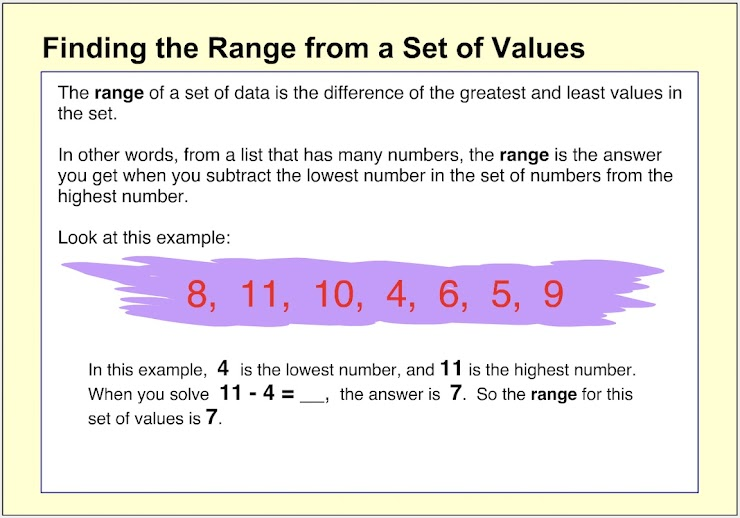Finding the Range Help Slide 2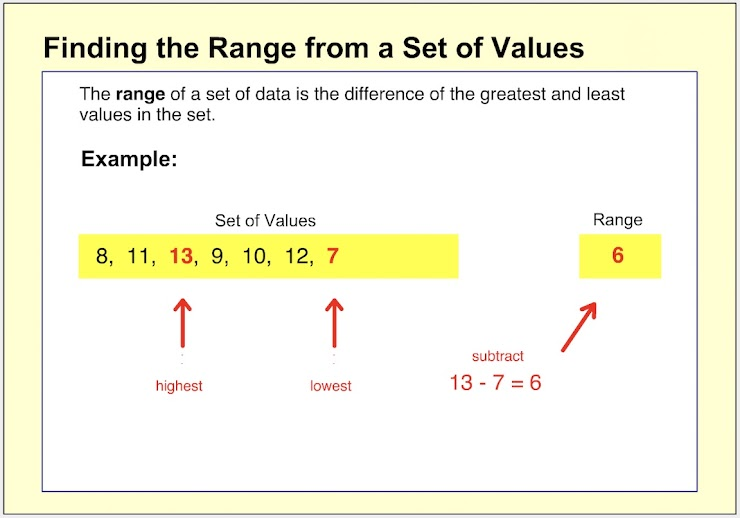Finding the Range Help Slide 3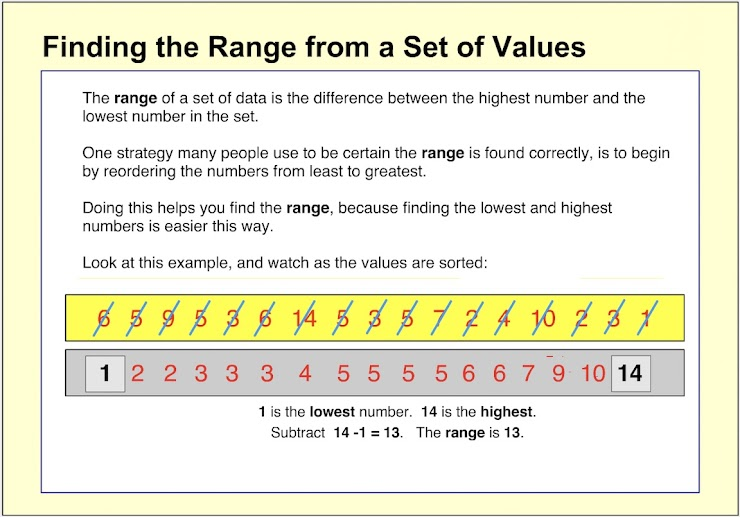Finding the Range Help Slide 4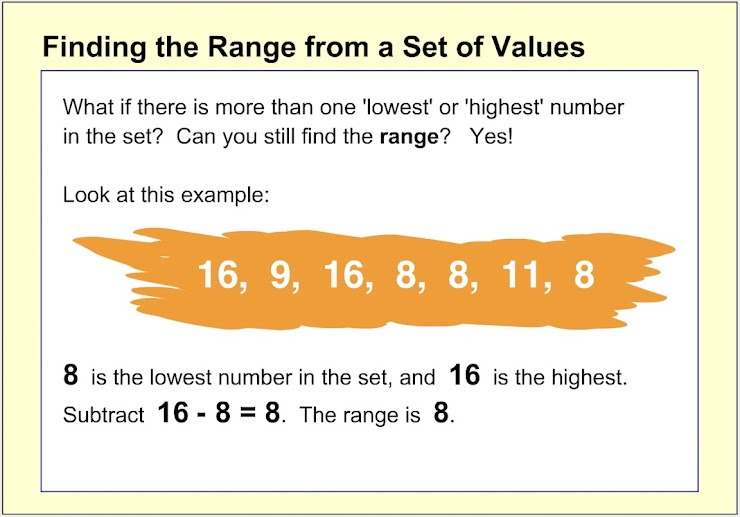Finding the Range Help Slide 5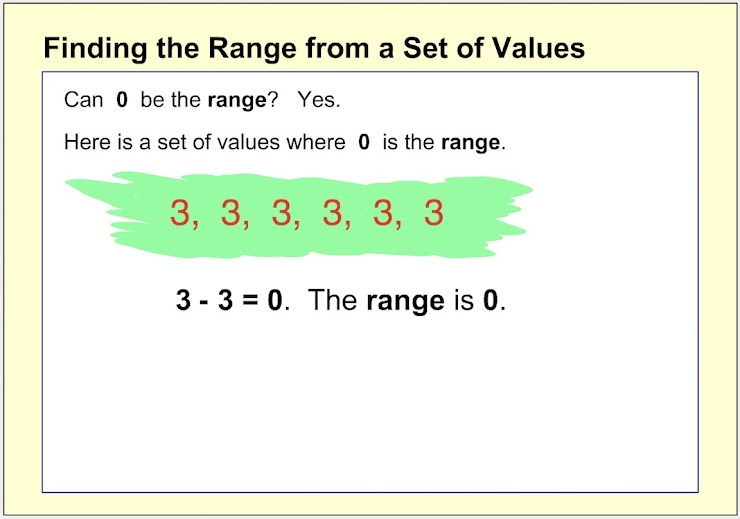1. Which choice below is the range for the set of values shown? *
1 point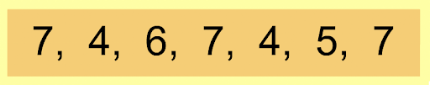Remember the range is found by finding the difference between the greatest and least value in the set of data.
2. Which choice below is the range for the set of values shown? *
1 point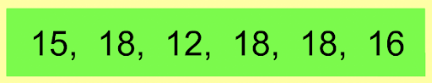3. Which choice below is the range for the set of values shown? *
1 point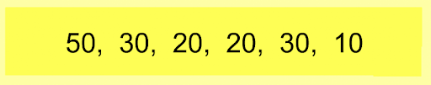4. Which choice below shows the correct equation to use to find the range? *
1 point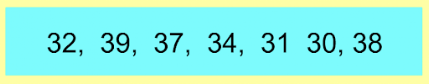5. On the line below, enter the range for the set of values shown. *
1 point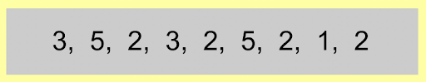6. On the line below, enter the range for the set of values shown. *
1 point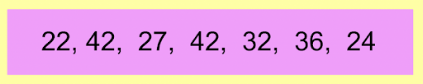7. On the line below, enter the range for the set of values shown. *
1 point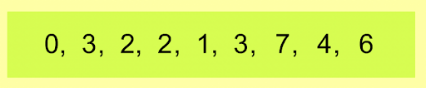8. On the line below, enter the range for the set of values shown. *
1 point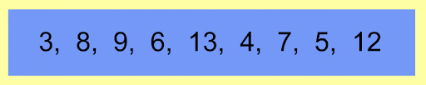9. On the line below, enter the range for the set of values shown. *
1 point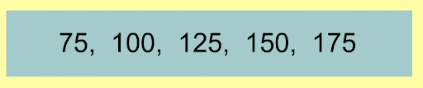10. On the line below, enter the range for the set of values shown. *
1 point